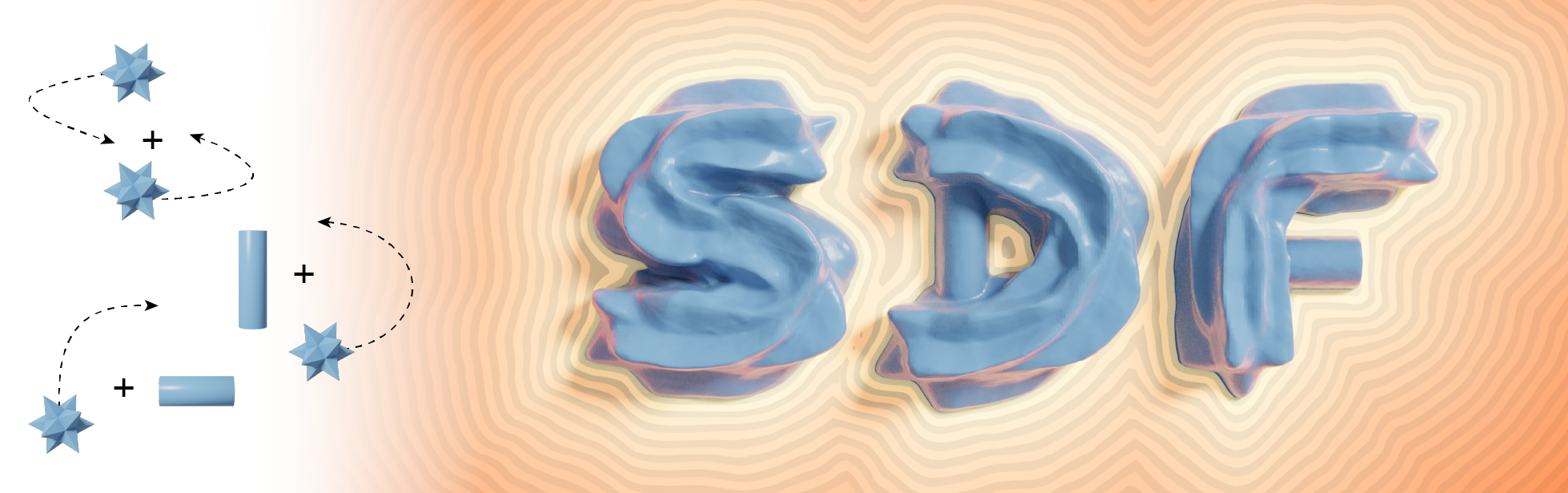# Constructive Solid Geometry on Neural Signed Distance Fields

Zoë Marschner, Massachusetts Institute of Technology and Carnegie Mellon University

Silvia Sellán, University of Toronto

Hsueh-Ti Derek Liu, Roblox Research and University of Toronto

Alec Jacobson, University of Toronto and Adobe ResearchOur method allows for the computation of exact neural SDFs of CSG operations. Here, we train one network to learn the swept volume of a stellated dodecahedron shape, parametric over the control points of the cubic Bézier path it is swept along. Specific swept volumes within this parameter space are then unioned together and with cylinders, resulting in a neural implicit which thanks to our regularization term forms an exact SDF of the word “SDF.”

## Abstract

Signed Distance Fields (SDFs) parameterized by neural networks have recently gained popularity as a fundamental geometric representation. However, editing the shape encoded by a neural SDF remains an open challenge. A tempting approach is to leverage common geometric operators (e.g., boolean operations), but such edits often lead to incorrect non-SDF outputs (which we call Pseudo-SDFs), preventing them from being used for downstream tasks. In this paper, we characterize the space of Pseudo-SDFs, which are eikonal yet not true distance functions, and derive the closest point loss, a novel regularizer that encourages the output to be an exact SDF. We demonstrate the applicability of our regularization to many operations in which traditional methods cause a Pseudo-SDF to arise, such as CSG and swept volumes, and produce a true (neural) SDF for the result of these operations.

## Closest Point Loss

Our work introduces the closest point loss, which can be used to penalize non-SDF regions of neural implicit functions. Unlike the previously used eikonal loss, our loss is able to penalize functions that are eikonal but do not obey the distance property (which we call Pseudo-SDFs).

The formulation of our loss is based off the closest point map, which for an exact SDF uses the distance value and gradient direction to project a point to the zero level set. In order to measure how much a given function deviates from an SDF, we sum the squared distance values of a set of test points after the map is applied.

## Results

With the closest point loss as a regularizer, we can create exact neural SDFs of CSG operations—and by adding a parameter space to our networks, we can solve whole families of these CSG problems by training a single network. This pin shape is created by performing unions and intersections between three primitives, the dimensions of which are controlled by four latent variables of the network. We visualize the different shapes our network outputs for different points in this latent space.